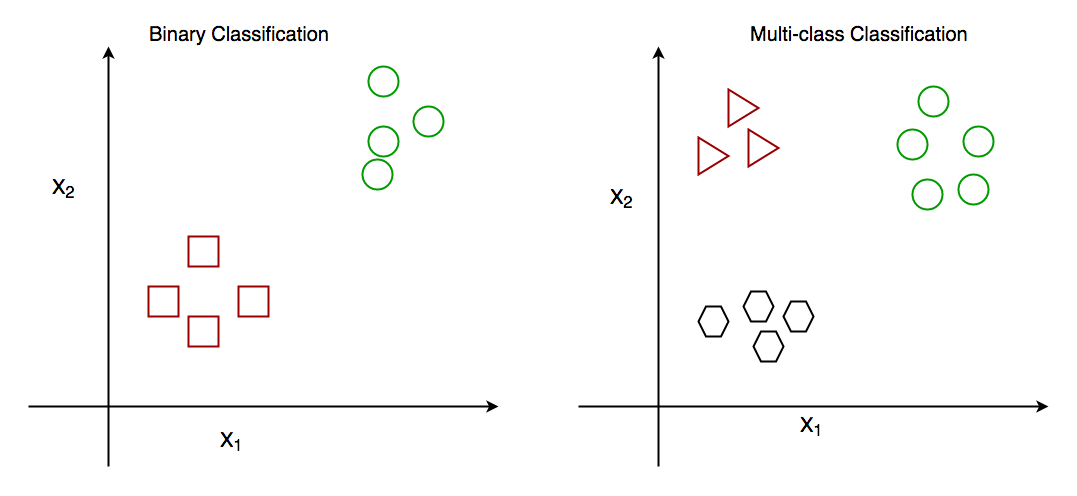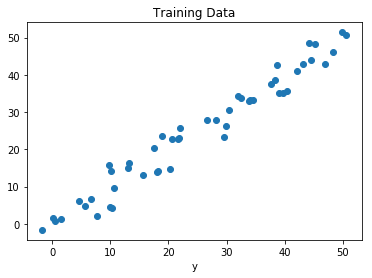# ML | Classification vs Regression

Prerequisite :Classification and Regression

Classification and Regression are two major prediction problems which are usually dealt with Data mining and machine learning.

Classification is the process of finding or discovering a model or function which helps in separating the data into multiple categorical classes i.e. discrete values. In classification, data is categorized under different labels according to some parameters given in input and then the labels are predicted for the data.
The derived mapping function could be demonstrated in the form of “IF-THEN” rules. The classification process deal with the problems where the data can be divided into binary or multiple discrete labels.

Let’s take an example, suppose we want to predict the possibility of the wining of match by Team A on the basis of some parameters recorded earlier. Then there would be two labels Yes and No.Fig : Binary Classification and Multiclass Classification

Regression is the process of finding a model or function for distinguishing the data into continuous real values instead of using classes or discrete values. It can also identify the distribution movement depending on the historical data. Because a regression predictive model predicts a quantity, therefore, the skill of the model must be reported as an error in those predictions
Let’s take a similar example in regression also, where we are finding the possibility of rain in some particular regions with the help of some parameters recorded earlier. Then there is a probability associated with the rain.Fig : Regression of Day vs Rainfall (in mm)

Comparison between Clasification and Regression:

Paramenter CLASSIFICATION REGRESSION
Basic Mapping Function is used for mapping of values to predefined classes. Mapping Function is used for mapping of values to continuous output.
Involves prediction of Discrete values Continuous values
Nature of the predicted data Unordered Ordered
Method of calculation by measuring accuracy by measurement of root mean square error
Example Algorithms Decision tree, logistic regression, etc. Regression tree (Random forest), Linear regression, etc.
My Personal Notes arrow_drop_upCheck out this Author's contributed articles.

If you like GeeksforGeeks and would like to contribute, you can also write an article using contribute.geeksforgeeks.org or mail your article to contribute@geeksforgeeks.org. See your article appearing on the GeeksforGeeks main page and help other Geeks.

Please Improve this article if you find anything incorrect by clicking on the "Improve Article" button below.

Improved By : Akanksha_Rai

Practice Tags :

5

Please write to us at contribute@geeksforgeeks.org to report any issue with the above content.